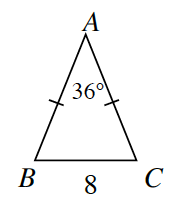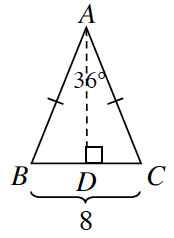### Home > GC > Chapter 7 > Lesson 7.2.3 > Problem7-68

7-68.

Tromika wants to find the area of the isosceles triangle below.1.She decided to start by drawing a height from vertex $A$ to side $\overline{BC}$ as shown at right. Will the two smaller triangles be congruent? In other words, is $ΔABD≅ΔACD$? Why or why not?

Look at the two triangles that are created. They share a side, and the ticks marks mean the sides are equal. How could they be congruent?

Yes, they are congruent by $HL≅$.

2. What is $m∠DAB$? $BD$?

If the segment (height) bisects both $∠BAC$ and segment $BC$, how can you calculate the measure of half of each?

$m∠DAB=18°$
$BD=4$

3. Find $AD$. Show how you got your answer.

Which trig ratio can you use to determine this length?

4. Find the area of $ΔABC$.

How do you find the area of a triangle? Do you have enough information to calculate the area?